# 8.4 Matrix Operations

•• Contributed by CK12
• CK12

Algebra refers to your ability to manipulate variables and unknowns based on rules and properties. Matrix algebra is extremely similar to the algebra you already know for numbers with a few important differences.  What are these differences?

## Algebra with Matrices

Two matrices of the same order can be added by summing the entries in the corresponding positions.

$$\left[\begin{array}{lll} 1 & 2 & 3 \\ 4 & 5 & 6 \end{array}\right]+\left[\begin{array}{lll} 6 & 5 & 4 \\ 3 & 2 & 1 \end{array}\right]=\left[\begin{array}{lll} 7 & 7 & 7 \\ 7 & 7 & 7 \end{array}\right]$$

Two matrices of the same order can be subtracted by subtracting the entries in the corresponding positions.

$$\left[\begin{array}{ccc}10 & 9 & 8 \\ 7 & 6 & 5\end{array}\right]-\left[\begin{array}{ccc}2 & 2 & 2 \\ 2 & 2 & 2\end{array}\right]=\left[\begin{array}{ccc}8 & 7 & 6 \\ 5 & 4 & 3\end{array}\right]$$

Multiplication

You can find the product of matrix $$A$$ and matrix $$B$$ if the number of columns in matrix $$A$$ matches the number of rows in matrix $$B$$. Another way to remember this is when you write the orders of matrix $$A$$ and matrix $$B$$ next to each other they must be connected by the same number. The resulting matrix has the number of rows from the first matrix and the number of columns from the second matrix.

$$(2 \times 3) \cdot(3 \times 5)=(2 \times 5)$$

To compute the first entry of the resulting $$2 \times 5$$ matrix you should match the first row from the first matrix and the first column of the second matrix. The arithmetic operation to combine these numbers is identical to taking the dot product between two vectors.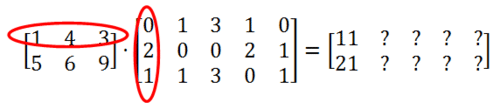• The entry in the first row first column of the new matrix is computed as $$1 \cdot 0+4 \cdot 2+3 \cdot 1=11$$.
• - The entry in the second row first column of the new matrix is computed as $$5 \cdot 0+6 \cdot 2+9 \cdot 1=21$$
• - The entry in the first row second column of the new matrix is computed as $$1 \cdot 1+4 \cdot 0+3 \cdot 1=4$$
• - The entry in the second row second column of the new matrix is computed as $$5 \cdot 1+6 \cdot 0+9 \cdot 1=14$$

Continue this pattern and you will find that the solution to this multiplication is:

C=$$\left[\begin{array}{ccccc} 11 & 4 & 12 & 9 & 7 \\ 21 & 14 & 42 & 17 & 15 \end{array}\right]$$

Other Properties of Matrix Algebra

• Commutativity holds for matrix addition. This means that when matrices $$A$$ and $$B$$ can be added (when they have matching orders), then: $$A+B=B+A$$
• Commutativity does not hold in general for matrix multiplication.
• Associativity holds for both multiplication and addition. $$(A B) C=A(B C),(A+B)+C=A+(B+C)$$
• Distribution over addition and subtraction holds. $$A(B \pm C)=A B \pm A C$$

## Examples

Example 1

Earlier, you were asked what the differences between matrix and regular algebra are. The main difference between matrix algebra and regular algebra with numbers is that matrices do not have the commutative property for multiplication. There are other complexities that matrices have, but many of them stem from the fact that for most matrices $$A B \neq B A$$.

Example 2

Show the commutative property does not hold by demonstrating $$A B \neq B A$$

$$A=\left[\begin{array}{ccc}0 & -1 & 8 \\ 1 & 2 & 0 \\ 4 & 3 & 12\end{array}\right], \quad B=\left[\begin{array}{ccc}1 & 5 & 1 \\ 2 & 2 & 1 \\ 4 & 3 & 0\end{array}\right]$$

$$A B=\left[\begin{array}{ccc}30 & 22 & -1 \\ 5 & 9 & 3 \\ 58 & 62 & 7\end{array}\right]$$

$$B A=\left[\begin{array}{ccc}9 & 12 & 20 \\ 6 & 5 & 28 \\ 3 & 2 & 32\end{array}\right]$$

Example 3

Compute the following matrix arithmetic: $$10 \cdot(2 A-3 C) \cdot B$$.

$$A=\left[\begin{array}{ll}1 & 2 \\ 4 & 5\end{array}\right], B=\left[\begin{array}{lll}0 & 1 & 2 \\ 4 & 3 & 2\end{array}\right], C=\left[\begin{array}{cc}12 & 0 \\ 1 & 3\end{array}\right]$$

When a matrix is multiplied by a scalar (such as with $$2 A$$ ), multiply each entry in the matrix by the scalar.

$$2 A=\left[\begin{array}{cc}2 & 4 \\ 8 & 10\end{array}\right]$$

$$-3 C=\left[\begin{array}{cc}-36 & 0 \\ -3 & -9\end{array}\right]$$

$$2 A-3 C=\left[\begin{array}{cc}-34 & 4 \\ 5 & 1\end{array}\right]$$

Since the associative property holds, you can either distribute the ten or multiply by matrix $$B$$ next.

$$(2 A-3 C) \cdot B=\left[\begin{array}{ccc}16 & -22 & -60 \\ 4 & 8 & 12\end{array}\right]$$

$$10 \cdot(2 A-3 C) \cdot B=\left[\begin{array}{ccc}160 & -220 & -600 \\ 40 & 80 & 120\end{array}\right]$$

Example 4

Use your calculator to input and compute the following matrix operations.

$$\begin{array}{l}A=\left[\begin{array}{ccc}54 & 65 & 12 \\ 235 & 322 & 167 \\ 413 & 512 & 123\end{array}\right], \quad B=\left[\begin{array}{ccc}163 & 212 & 466 \\ 91 & 221 & 184 \\ 42 & 55 & 42\end{array}\right] \\ A^{T} \cdot B \cdot A-100 A & \end{array}$$

Most graphing calculators like the TI-84 can do operations on matrices. Find where you can enter matrices and enter the two matrices.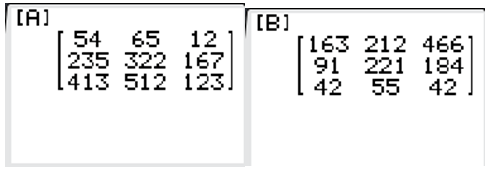Then type in the appropriate operation and see the result.  The TI-84 has a built in Transpose button.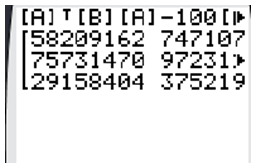The actual numbers on this guided practice are less important than the knowledge that your calculator can perform all of the matrix algebra demonstrated in this concept.  It is useful to fully know the capabilities of the tools at your disposal, but it should not replace knowing why the calculator does what it does.

Example 5

Matrix multiplication can be used as a transformation in the coordinate system.  Consider the triangle with coordinates (0, 0) (1, 2) and (1, 0) the following matrix:

\left[\begin{array}{cc}

\cos 90^{\circ} & \sin 90^{\circ} \\

-\sin 90 & \cos 90

\end{array}\right]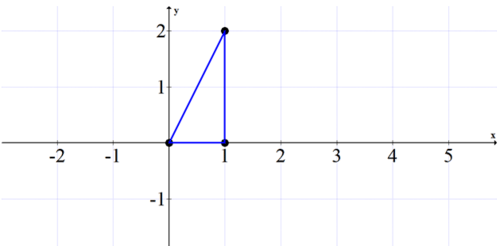What does the new picture look like?

The matrix simplifies to become:

$$\left[\begin{array}{ll}\cos 90^{\circ} & \sin 90^{\circ} \\ -\sin 90 & \cos 90\end{array}\right]=\left[\begin{array}{cc}0 & 1 \\ -1 & 0\end{array}\right]$$

When applied to each point as a transformation, a new point is produced. Note that $$\left[\begin{array}{ll}x & y\end{array}\right]$$ is a matrix representing each original point and $$\left[x^{\prime} y^{\prime}\right]$$ is the new point. The $$x^{\prime}$$ is read as $${ }^{a} x$$ prime" and is a common way to refer to a result after a transformation.

$$\left[\begin{array}{ll}x & y\end{array}\right] \cdot\left[\begin{array}{cc}0 & 1 \\ -1 & 0\end{array}\right]=\left[\begin{array}{ll}x^{\prime} & y^{\prime}\end{array}\right]$$

$$\left[\begin{array}{ll}0 & 0\end{array}\right] \cdot\left[\begin{array}{cc}0 & 1 \\ -1 & 0\end{array}\right]=\left[\begin{array}{ll}0 & 0\end{array}\right]$$

$$\left[\begin{array}{ll}1 & 2\end{array}\right] \cdot\left[\begin{array}{cc}0 & 1 \\ -1 & 0\end{array}\right]=\left[\begin{array}{ll}-2 & 1\end{array}\right]$$

$$\left[\begin{array}{ll}1 & 0\end{array}\right] \cdot\left[\begin{array}{cc}0 & 1 \\ -1 & 0\end{array}\right]=\left[\begin{array}{ll}0 & 1\end{array}\right]$$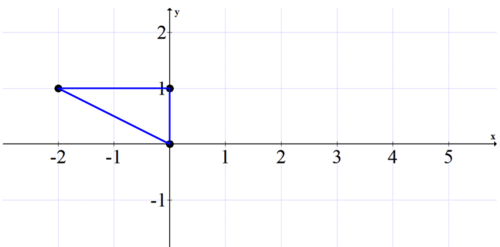Notice how the matrix transformation rotates graphs in a counterclockwise direction $$90^{\circ}$$.

$$\left[\begin{array}{ll}x & y\end{array}\right]\left[\begin{array}{ll}\cos 90^{\circ} & \sin 90^{\circ} \\ -\sin 90 & \cos 90\end{array}\right]=\left[\begin{array}{ll}-y & x\end{array}\right]$$

The matrix transformation applied in the following order will rotate a graph clockwise $$90^{\circ}$$.

$$\left[\begin{array}{cc}\cos 90^{\circ} & \sin 90^{\circ} \\ -\sin 90 & \cos 90\end{array}\right]\left[\begin{array}{l}x \\ y\end{array}\right]=\left[\begin{array}{c}y \\ -x\end{array}\right]$$

Review

$$A=\left[\begin{array}{ll}2 & 7 \\ 3 & 8\end{array}\right], B=\left[\begin{array}{lll}0 & 5 & 1 \\ 3 & 4 & 6\end{array}\right], C=\left[\begin{array}{cc}14 & 6 \\ 1 & 2\end{array}\right], D=\left[\begin{array}{ll}5 & 0 \\ 1 & 2\end{array}\right]$$

1. Find $$A C$$. If not possible, explain.

2. Find $$B A$$. If not possible, explain.

3. Find $$C A$$. If not possible, explain.

4. Find $$4 B^{T}$$. If not possible, explain.

5. Find $$A+C$$. If not possible, explain.

6. Find $$D-A$$. If not possible, explain.

7. Find $$2(A+C-D)$$. If not possible, explain.

8. Find $$(A+C) B$$. If not possible, explain.

9. Find $$B(A+C)$$. If not possible, explain.

10. Show that $$A(C+D)=A C+A D$$

11. Show that $$A(C-D)=A C-A D$$.

Practice using your calculator for #12-#15.

$$E=\left[\begin{array}{ccc}312 & 59 & 34 \\ 342 & 156 & 189 \\ 783 & 23 & 133\end{array}\right], F=\left[\begin{array}{ccc}33 & 72 & 21 \\ 93 & 41 & 94 \\ 62 & 75 & 72\end{array}\right], G=\left[\begin{array}{ccc}11 & 735 & 67 \\ 93 & 456 & 2 \\ 94 & 34 & 0\end{array}\right]$$

12. Find $$E+F+G$$

13. Find $$2 E$$

14. Find $$4 F$$

15. Find $$(E+F) G$$